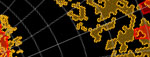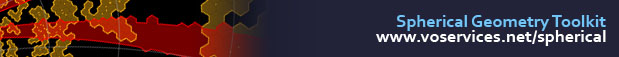## Learn by Examples

These simple examples are here to guide you on your first journey into programming with halfspaces, convexes and regions as well as the HTM both in C# and SQL. Are we missing something basic? Please let us know today!

### C#

Points on the sphere are represented as 3D unit vectors. You can create one using the (x,y,z) coordinates or even (RA, Dec) as in the following example:

 ``` Cartesian p1 = new Cartesian(180, 0); Cartesian p2 = new Cartesian(181, 0); ```

Halfspaces are simplest contructs that can specify spherical caps of any direction and size. They are defined by the center point and the radius. Here we create two using the previous points and build a convex that represents their intersections:

 ``` double theta = Math.PI / 180; // 1 degree radii Halfspace h1 = new Halfspace(p1, Math.Cos(theta)); Halfspace h2 = new Halfspace(p2, Math.Cos(theta)); Convex c = new Convex(); c.Add(h1); c.Add(h2); ```

A region is a union of convexes. Here we define one using two convexes. The simplification step creates an irreducible representation and calculates the analytic area:

 ``` Region r = new Region(); r.Add(c1); r.Add(c2); r.Simplify(); Console.Out.WriteLine(r.Area); ```

The toolkit provides the full Boolean algebra for regions. Below is how you can create the union of two regions:

 ``` r.Union(r2); // regions may not be simplified r.Simplify(); // simplification from scratch Console.Out.WriteLine(r.Area); ```

An even better way is the following that makes use of the fully simplified representations:

 ``` r.SmartUnion(r2); // both simplified Console.Out.WriteLine(r.Area); ```

Calculating the HTM index of a point is as easy as the function call:

 ``` Cartesian p = new Cartesian(180,0); // Point on the sky Int64 htmID = Trixel.CartesianToHid20(p); // and its ID ```

Another function returns the vertices of a given trixel:

 ``` Cartesian v1, v2, v3; // Vertices of the trixel Trixel.ToTriangle(htmID, out v1, out v2, out v3); ```

The HTM cover of a region is an approximate description for speeding up searches. These covers can be completely inside the region (`Markup.Inner`) or completely cover it (`Markup.Outer`) Instantiate a cover object to investigate its properties as in this example:

 ``` Cover k = new Cover(region); k.Run(); // Default processing, see also k.Step() List inner = k.GetTrixels(Markup.Inner); List outer = k.GetTrixels(Markup.Outer); ```

We current do not supply ready-to-use indexing mechanisms that implement the actual search pattern but this is fairly straighforward to do. We expect that programmers will use specific solutions that are most suited for their data structures. Better yet, use a database engine with proper indices.

### SQL

The easiest way to create simple regions is to use our region specification language, which is also available for .NET programmers via the parser in the Shape namespace. The description follows a few simple rules that can be deduced from the following examples but we also have a BNF specification available for the purists in the documentation. Here is how to create regions in SQL using these strings, create their unions and return their areas:

 ``` DECLARE @s VARCHAR(MAX), @r VARBINARY(MAX),         @z VARCHAR(MAX), @u VARBINARY(MAX) SELECT @s = 'REGION CIRCLE J2000 180 0 60',        @z = 'POLY J2000 180 0 182 0 182 2 180 2',        @r = sph.fSimplifyString(@s),        @u = sph.fUnion(@r,sph.fSimplifyString(@z)) SELECT sph.fGetArea(@r), SELECT sph.fGetArea(@u) -- Returns: 3.14151290574491 6.35572804450646 ```

One of the most basic HTM tasks is to compute the HTM addresses or `HtmID` for all entries of a table. The following simple command assumes that the specified table was defined with the appropriate HTM column and populates that using the (RA, Dec) positions that are also stored for each row:

 ``` UPDATE PhotoObj SET HtmID = dbo.fHtmEq(RA,Dec) ```

The query below is perhaps the most advanced of them all. It creates the HTM cover for a given region on the fly and uses the resulting ranges to filter the catalog. While this will overshoot and return more than what is covered by the specified region, it will execute very fast. The actual geometry cut can be done in subsequent steps or even written into the same query, but we leave that out here for simplicity.

 ``` WITH Cover AS (     SELECT * FROM dbo.fHtmCoverRegion       ('REGION CIRCLE J2000 180 0 10') ) SELECT o.ObjID FROM PhotoObj AS o INNER JOIN Cover AS c     ON o.HtmID BETWEEN c.HtmIDStart AND c.HtmIDEnd ```

Simple, isn't it? Why don't you try it now and let us know how it goes. Enjoy!Copyright © 2003-2010 Johns Hopkins University, Department of Physics & Astronomy Release: 3.0